﻿ 飞机冲压空气涡轮系统的动态特性分析Download PDF文章快速检索 高级检索

1. 航空工业金城南京机电液压工程研究中心, 南京 211106;
2. 航空机电系统综合航空科技重点实验室, 南京 211106

Analysis of dynamic behavior of aircraft ram air turbine system
XIA Tianxiang1,2, LU Yueliang1,2, KE Bing1,2
1. AVIC Jincheng Nanjing Engineering Institute of Aircraft System, Nanjing 211106, China;
2. Aviation Science and Technology Key Laboratory of Aviation Mechanical and Electrical System, Nanjing 211106, China
Abstract: The ram air turbine system is a commonly used airborne auxiliary power system for emergency situations. Aiming at the Ram Air Turbine (RAT) system, the physical architecture is firstly constructed based on the working principle. On the basis of the physical architecture, the mechanical analysis is conducted for turbine, energy conversion unit, actuator, and deployment mechanism components. Finally, based on the mechanical equations, a multidisciplinary model of the RAT involving mechanics, hydraulics and multi-body dynamics is built. This system model is used to simulate the dynamic behaviors under different working and design conditions and the influences on performances of key design parameters were studied for forward design procedure. Finally, the results of a wind tunnel test verified the accuracy of the system model.
Keywords: ram air turbine    system model    multidisciplinary simulation    dynamic behavior    wind tunnel test

1 系统组成及工作原理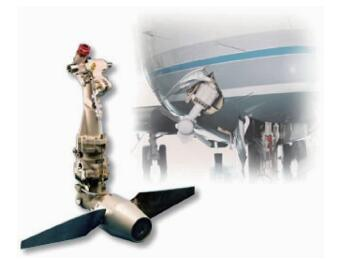图 1 空客A330的RAT外形 Fig. 1 Appearance of RAT on A330 airplane
2 系统数学模型 2.1 涡轮部件和能源转换装置

RAT工作时，气流作用在涡轮叶片上，产生绕涡轮轴线的驱动力矩MD和绕叶片转轴的扭转力矩MT。由于能源转换装置存在额定转速限制，涡轮部件内设计有调速机构以对转速进行被动控制。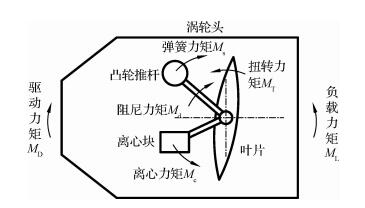图 2 涡轮力矩 Fig. 2 Moments on turbine

 ${M_{\rm{T}}} + {M_{\rm{c}}} - {M_{\rm{s}}} - {M_{\rm{d}}} = {J_1}\ddot \theta$ （1）
 ${M_{\rm{D}}} - {M_{\rm{L}}} = {J_2}\dot \omega$ （2）

1) 扭转力矩MT和驱动力矩MD

 ${M_{\rm{T}}} = {T_{\rm{T}}}\left( {\lambda , \theta } \right){P_{\rm{d}}} = {T_{\rm{T}}}\left( {\lambda , \theta } \right){\rm{ \mathsf{ π} }}{\rho _{{\rm{air}}}}{V^2}R_{\rm{T}}^3$ （3）
 ${M_{\rm{D}}} = {T_{\rm{D}}}\left( {\lambda , \theta } \right){P_{\rm{d}}} = {T_{\rm{D}}}\left( {\lambda , \theta } \right){\rm{ \mathsf{ π} }}{\rho _{{\rm{air}}}}{V^2}R_{\rm{T}}^3$ （4）
 $V = {V_0}\sin \phi$ （5）

2) 离心力矩Mc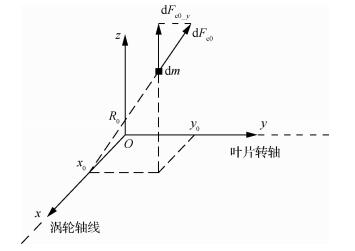图 3 叶片组件质量微元坐标系 Fig. 3 Coordinate system of infinitesimal masson the blade component
 ${R_0} = \sqrt {y_0^2 + z_0^2}$ （6）

 ${\rm{d}}{F_{{\rm{c}}0}} = {\rm{d}}m{\omega ^2}{R_0}$ （7）

 ${\rm{d}}{F_{{\rm{c0\_}}y}} = {\rm{d}}{F_{{\rm{c0}}}}\frac{{{z_0}}}{{{R_0}}} = {\omega ^2}{z_0}{\rm{d}}m$ （8）

 ${\rm{d}}{M_{{\rm{c0\_}}y}} = {\rm{d}}{F_{{\rm{c0\_}}y}}{x_0} = {\omega ^2}{x_0}{z_0}{\rm{d}}m$ （9）

 ${M_{\rm{c}}} = \int {{\rm{d}}{M_{{\rm{c0\_}}y}}} = \int {{\rm{d}}m{\mkern 1mu} {\omega ^2}{x_0}{z_0}} = {\omega ^2}\int {{\rm{d}}m{\mkern 1mu} {x_0}{z_0}}$ （10）

3) 弹簧力矩Ms和阻尼力矩Md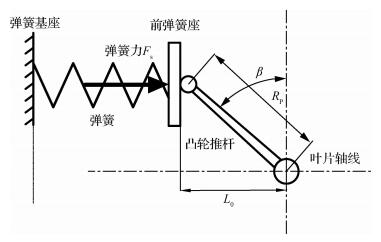图 4 凸轮示意图 Fig. 4 Diagrammatic sketch of cam

 $\begin{array}{l} {F_{\rm{s}}} = 0.5\left( {k\Delta x + {F_{{\rm{s0}}}}} \right) = 0.5k\left( {{R_{\rm{P}}}\cos \beta - {L_0}} \right) + \\ \;\;\;\;\;0.5{F_{{\rm{s0}}}} \end{array}$ （11）
 ${M_{\rm{s}}} = {F_{\rm{s}}}{R_{\rm{P}}}\cos \beta$ （12）

 ${M_{\rm{d}}} = C\dot \theta$ （13）

4) 负载力矩ML

 ${M_{\rm{L}}} = \frac{{{P_{\rm{L}}}}}{\omega } = \frac{{{P_{\rm{G}}}/{\eta _{\rm{G}}} + qp/{\eta _{\rm{P}}}}}{{{\eta _{\rm{C}}}\omega }}$ （14）

2.2 展开装置

1) 展开力Fe

 ${F_{\rm{e}}}\left( t \right) = {k_{\rm{e}}}\left( {{x_{{\rm{e}}0}} - {x_{\rm{e}}}\left( t \right)} \right)$ （15）

2) 液压缓冲力Fb

 $Q = {C_{\rm{d}}}A\sqrt {\frac{{2\left( {{P_{{\rm{in}}}} - {P_{{\rm{out}}}}} \right)}}{{{\rho _{{\rm{oil}}}}}}}$ （16）

 ${P_{{\rm{in}}}} = \frac{{{Q^2}\rho _{{\rm{oil}}}^2}}{{2C_{\rm{d}}^2{A^2}}} + {P_{{\rm{out}}}}$ （17）

 ${F_{\rm{b}}} = {P_{{\rm{in}}}}S$ （18）
2.3 展开随动机构

RAT展开过程中，展开装置外缸、内缸相互滑动，驱动支撑臂绕转轴向机体外旋转。此时，可将RAT看作一个连杆机构，如图 5所示。此外，RAT还设置有用于推开RAT舱门的随动机构，即舱门连杆。它通过两端的球铰分别与支撑臂和舱门结构连接。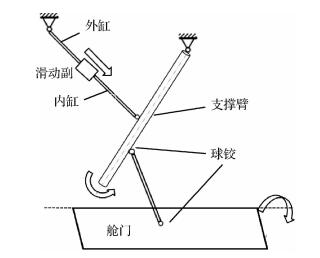图 5 RAT展开随动机构示意图 Fig. 5 Diagrammatic sketch of RAT deploymentmechanism component

3 系统多学科仿真建模

AMESim是机电一体化系统的多学科领域复杂系统建模仿真工具，适合建立复杂的多学科领域的系统模型，并在此基础上进行仿真计算和深入的元件或系统的稳态和动态性能分析。

3.1 涡轮部件和能源转换装置模型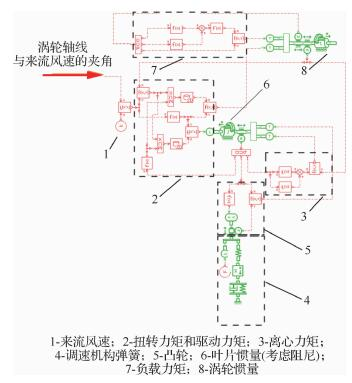图 6 涡轮部件和能源转换装置AMESim模型 Fig. 6 AMESim model of turbine and energyconversion unit
3.2 展开装置模型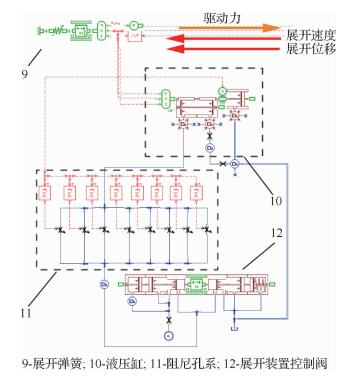图 7 展开装置AMESim模型 Fig. 7 AMESim model of actuator
3.3 随动机构模型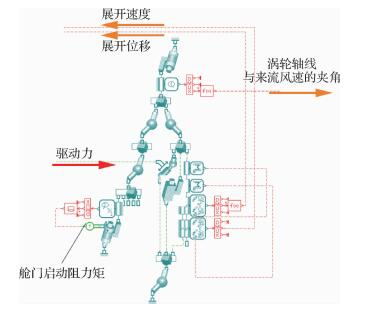图 8 随动机构AMESim模型 Fig. 8 AMESim model of deployment mechanismcomponent
4 仿真分析及试验

 Parameter Value Air density ρair/ (kg·m-3) 1.23 Turbine radius RT/mm 320 Wind speed V/(km·h-1) 245 Product of inertia Ixz/(kg·mm2) 107.6 Spring stiffness k/(N·mm-1) 190 Spring preload F0/N 2 500 Damping coefficient C/(N·s·m-1) 1.54 Discharge of pump q/(L·min-1) 2 Deployment spring stiffness ke/(N·mm-1) 20 Deployment spring preload xe0/mm 120 Distance between spherical joint and revolvingshaft S/mm 300
4.1 桨矩角稳定性分析

1) 风速对桨矩角稳定性的影响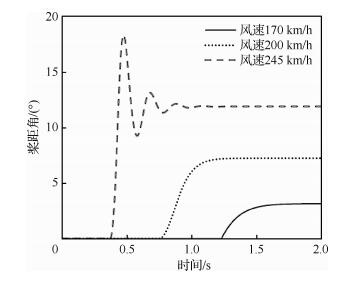图 9 不同风速下的桨矩角变化情况 Fig. 9 Variation of pitch angle under different windvelocities

2) 调速弹簧刚度对桨矩角稳定性的影响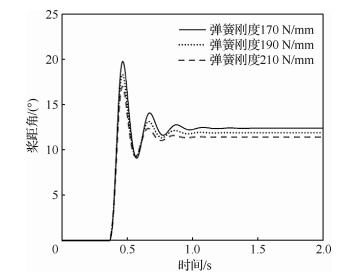图 10 不同调速弹簧刚度下的桨矩角变化情况 Fig. 10 Variation of pitch angle under different springstiffness

3) 调速弹簧初始弹力对桨矩角稳定性的影响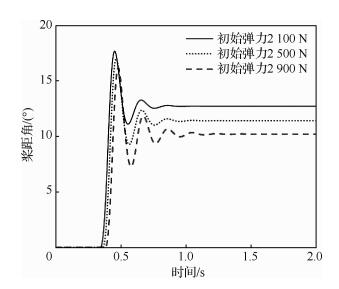图 11 不同调速弹簧初始弹力下的桨矩角变化情况 Fig. 11 Variation of pitch angle under different initialspring preloads

4) 调速机构阻尼系数对桨矩角稳定性的影响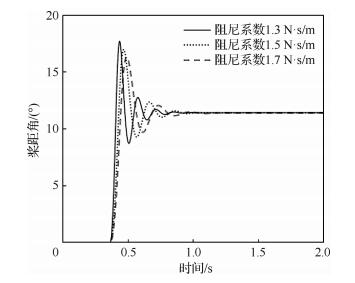图 12 不同阻尼系数下的桨矩角变化情况 Fig. 12 Variation of pitch angle under differentdamping coefficients
4.2 涡轮转速特性分析

RAT涡轮转速与功率输出和结构安全密切相关。调速机构的主要功能是确保转速在不同风速和负载功率下保持稳定。

1) 风速对涡轮转速特性的影响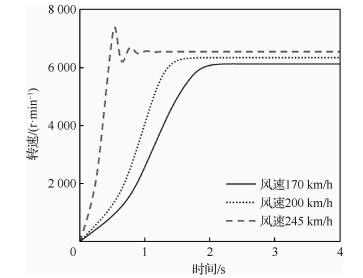图 13 不同风速下的转速变化情况 Fig. 13 Variation of rotation speed at different windvelocities

2) 负载对涡轮转速特性的影响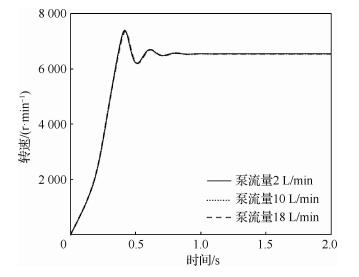图 14 不同泵流量下的转速变化情况 Fig. 14 Variation of rotation speed under differentpump discharges

3) 调速弹簧刚度对涡轮转速特性的影响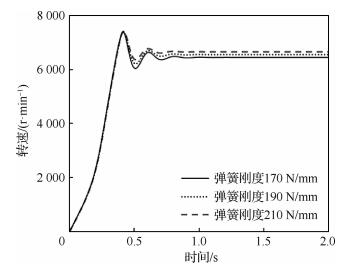图 15 不同调速弹簧刚度下的转速变化情况 Fig. 15 Variation of rotation speed under differentspring stiffness

4) 调速弹簧初始弹力对涡轮转速特性的影响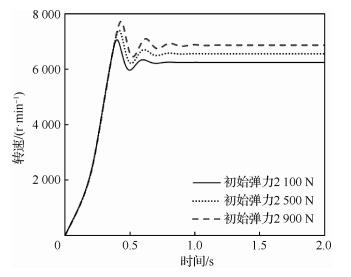图 16 不同调速弹簧初始弹力下的转速变化情况 Fig. 16 Variation of rotation speed under differentspring preloads

5) 调速机构阻尼系数对涡轮转速特性的影响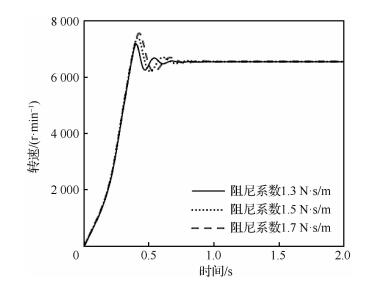图 17 不同阻尼系数下的转速变化情况 Fig. 17 Variation of rotation speed under differentdamping coefficients
4.3 展开时间分析

RAT展开时间是指从展开装置解锁到支撑臂展开到位的时间，与下节中的建转时间有所区别。展开时间主要由展开装置性能决定。

1) 展开装置弹簧刚度和预压缩量对展开时间的影响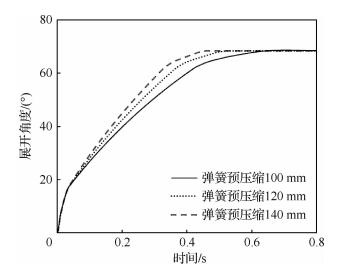图 18 不同展开装置弹簧预压缩下的展开角变化情况 Fig. 18 Variation of deployment angle underdifferent initial spring compression

2) 阻尼孔面积对展开时间的影响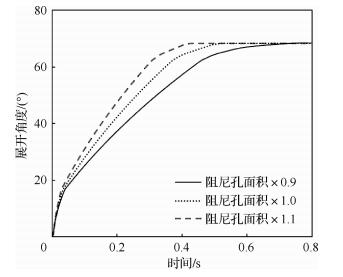图 19 不同阻尼系面积下的展开角变化情况 Fig. 19 Variation of deployment angle underdifferent orifice areas
4.4 试验分析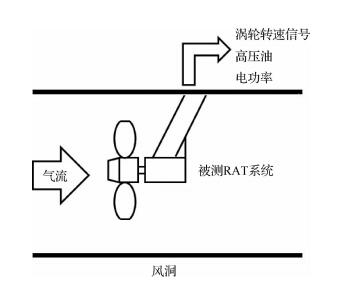图 20 风洞试验原理图 Fig. 20 Schematic diagram of wind tunnel test

 No. 1 2 3 4 5 6 7 8 Wind speed/(km·h-1) 170 170 200 200 200 245 245 245 Discharge of pump/(L·min-1) 2 10 2 10 18 2 18 18 Generator power/kW 0 0 0 0 0 0 0 7.5 Measured rotation speed/(r·min-1) 6 040 5 482 6 422 6 500 6 200 6 718 6 537 6 491 Calculated rotation speed/(r·min-1) 6 093 6 022 6 261 6 215 6 164 6 551 6 443 6 437 Error of rotation speed/% 0.88 9.85 -2.51 -4.38 -0.58 -2.47 -1.44 -0.83 Measured starting time/s 1.63 1.76 1.29 1.24 1.25 0.83 0.85 0.88 Calculated starting time/s 1.38 1.42 1.04 1.06 1.06 0.75 0.75 0.74 Error of starting time/% -15.34 -19.15 -19.38 -14.52 -15.20 -9.64 -11.76 -14.77

 $相对误差 = \frac{{计算值 - 试验值}}{{试验值}} \times 100\%$ （19）

1) 仿真得到的涡轮转速与实测值较为接近，所有工况的计算误差均在±10%以内(绝大部分误差在±5%以内)。此外，相对误差随转速的增加由正值转变为负值，且误差绝对值有减小的趋势。这是因为随着气动力的增加，摩擦力、阻尼力等无法准确计算的阻力影响比重逐渐降低；而由于实际产品各运动副均存在润滑，因此阻力真实值通常小于估算值。

2) 建转时间计算误差在-15%左右，在可接受范围内。实际工作中，建转时间的影响因素非常复杂，计算误差主要来源有：① RAT接受展开信号后，其展开解锁机构(包括解锁电磁铁、锁活塞等)存在响应时间。由于本模型未考虑此时间，导致计算值均小于测量值(即相对误差为负)；②真实RAT由于加工精度、液压油等因素影响，模型质量特性设置存在误差；③ RAT各运动副均存在摩擦力和阻尼，且难以准确确定。

5 结论

1) 当气流速度足够大且负载功率小于RAT最大功率时，风速对冲压空气涡轮系统涡轮转速的影响较大，而负载几乎不影响转速。

2) 增加调速机构弹簧刚度或阻尼系数能够增加转速稳定性；增加调速机构初始弹力能增加稳定转速，但不影响稳定性。

3) 增加展开装置弹簧刚度和预压缩量可以加速冲压空气涡轮系统展开；减小阻尼孔面积则会减缓展开。

  孔祥浩, 张卓然, 陆嘉伟, 等. 分布式电推进飞机电力系统研究综述[J]. 航空学报, 2018, 39(1): 021651. KONG X H, ZHANG Z R, LU J W, et al. Review of electric power system of distributed electric propulsion aircraft[J]. Acta Aeronautica et Astronautica Sinica, 2018, 39(1): 021651. (in Chinese) Cited By in Cnki (27) | Click to display the text  李永锋. 宽体客机飞控电作动系统设计[J]. 航空学报, 2017, 38(S1): 721531. LI Y F. Electrically powered actuation system design for long range wide body commercial aircraft[J]. Acta Aeronautica et Astronautica Sinica, 2017, 38(S1): 721531. (in Chinese) Cited By in Cnki (3) | Click to display the text  王莉, 戴泽华, 杨善水, 等. 电气化飞机电力系统智能化设计研究综述[J]. 航空学报, 2019, 40(2): 522405. WANG L, DAI Z H, YANG S S, et al. Review of intelligent design of electrified aircraft power systems[J]. Acta Aeronautica et Astronautica Sinica, 2019, 40(2): 522405. (in Chinese) Cited By in Cnki (1) | Click to display the text  MARE J C, FU J. Review on signal-by-wire and power-by-wire actuation for more electric aircraft[J]. Chinese Journal of Aeronautics, 2017, 30(3): 857-870. Click to display the text  BANNON D G, SEIDEL W E. Ram Air Turbine speed sensing ball detent over-speed prevention mechanism: US, 11148040[P]. 2005-06-08.  SWEARINGEN P, MARKS S J. Automatic ram air turbine deployment: US, 13052797[P]. 2011-03-21.  BAUER R P, STEVENS C G. Load shedding circuit for Ram Air Turbine: US, 13232199[P]. 2011-09-14.  王岩, 殷亚峰, 陈金华, 等. 冲压空气涡轮泵的温控节流孔计算方法[J]. 北京航空航天大学学报, 2017, 43(7): 1287-1292. WANG Y, YIN Y F, CHEN J H, et al. A calculation method for temperature control orifice of ram air turbine pump[J]. Journal of Beijing University of Aeronautics and Astronautics, 2017, 43(7): 1287-1292. (in Chinese) Cited By in Cnki (1) | Click to display the text  李兴利, 侯瑞, 周晓春. 冲压空气涡轮舱门开缝问题研究[J]. 航空科学技术, 2015, 26(12): 16-19. LI X L, HOU R, ZHOU X C. Research on clearance of RAT cabin door[J]. Aeronautical Science and Technology, 2015, 26(12): 16-19. (in Chinese) Cited By in Cnki | Click to display the text  王永鑫, 崔宇. 冲压空气涡轮在民用涡桨飞机中的应用[J]. 航空制造技术, 2014(14): 86-88. WANG Y X, CUI Y. Application of ram air turbine in civil turbo-prop aircraft[J]. Journal of Aeronautical Manufacturing Technology, 2014(14): 86-88. (in Chinese) Cited By in Cnki (6) | Click to display the text  WANG J. Research on several similarity criteria of ram air turbine operating under high-low altitude[C]//2016 IEEE/CSAA International Conference on Aircraft Utility Systems, 2016: 88-92.  张冬雨, 杨斐, 翟笑天, 等. 冲压空气涡轮气动性能快速算法研究[J]. 江苏航空, 2016(1): 4-6. ZHANG D Y, YANG F, ZHAI X T, et al. Research on aerodynamic performance of ram air turbine[J]. Jiangsu Aeronautics, 2016(1): 4-6. (in Chinese)  刘思永, 方祥军, 王屏. 可调桨距冲压空气涡轮气动特性实验与数值分析[J]. 航空动力学报, 2003(5): 587-592. LIU S Y, FANG X J, WANG P. The aerodynamic characteristic experiment and numerical analysis of ram air turbine with auto coarse pitch[J]. Journal of Aerospace Power, 2003(5): 587-592. (in Chinese) Cited By in Cnki (11) | Click to display the text  吴佳.某冲压空气涡轮结构设计及强度寿命振动分析[D].南京: 南京航空航天大学, 2008. WU J. Research on structure design and strength fatigue life vibration Analysis of a typical ram air turbine[D]. Nanjing: Nanjing University of Aeronautics and Astronautics, 2008 (in Chinese).  马树微, 李静琳, 陈曦, 等. 多级固体运载火箭分级多学科设计优化[J]. 北京航空航天大学学报, 2016, 42(3): 542-550. MA S W, LI J L, CHEN X, et al. Stage-wise multidisciplinary design optimization for multi-stage solid launch vehicle[J]. Aeronautical Science and Technology, 2016, 42(3): 542-550. (in Chinese) Cited By in Cnki (5) | Click to display the text  柏艳红, 陈聪, 孙志毅, 等. 基于AMESim的电液阀控缸系统线性化分析[J]. 系统仿真学报, 2014, 26(7): 1430-1434. BAI Y H, CHEN C, SUN ZH Y, et al. Linear analysis of electro-hydraulic valve-controlled cylinder system based on AMESim[J]. Journal of System Simulation, 2014, 26(7): 1430-1434. (in Chinese) Cited By in Cnki (28) | Click to display the text  黄大伟, 陈新元, 张俊峰, 等. 泵车臂架随动控制研究及其联合仿真[J]. 系统仿真学报, 2014, 26. HUANG D W, CHEN X Y, ZHANG J F, et al. Research and co-simulation on follow-up control for concrete pump truck[J]. Journal of System Simulation, 2014, 26. (in Chinese) Cited By in Cnki (2) | Click to display the text  陈永琴, 汪天兴, 苏三买, 等. 反推力装置液压作动系统AMESim建模与仿真[J]. 航空动力学报, 2017(11): 238-246. CHEN Y Q, WANG T X, SU S M, et al. Modeling and simulation of thrust reverser hydraulic actuation system based on AMESim[J]. Journal of Aerospace Power, 2017(11): 238-246. (in Chinese) Cited By in Cnki (2) | Click to display the text  周兰午. 风力机离心调速系统特性分析[J]. 河北机电学院学报, 1990, 2(1): 1-7. ZHOU L W. Analysis of the stability of a centrifugal regulator for wind mills[J]. Journal of Hebei Mechanical Engineering College, 1990, 2(1): 1-7. (in Chinese)  江桂云, 王勇勤, 严兴春, 等. 基于小孔节流的静压油膜轴承动态特性分析[J]. 机械设计, 2014, 31(7): 64-69. JIANG G Y, WANG Y Q, YAN X C, et al. Dynamic characteristics analysis on hydrostatic oil film bearing with orifice restrictor[J]. Journal of Machine Design, 2014, 31(7): 64-69. (in Chinese) Cited By in Cnki (19) | Click to display the text
http://dx.doi.org/10.7527/S1000-6893.2019.23242

0

#### 文章信息

XIA Tianxiang, LU Yueliang, KE Bing

Analysis of dynamic behavior of aircraft ram air turbine system

Acta Aeronautica et Astronautica Sinica, 2020, 41(3): 423242.
http://dx.doi.org/10.7527/S1000-6893.2019.23242